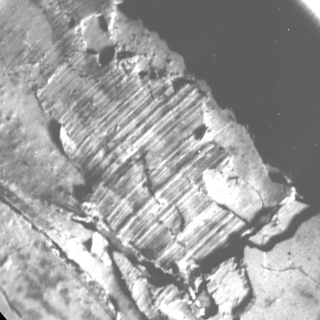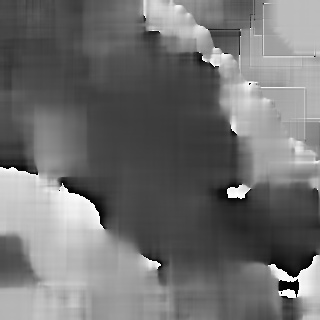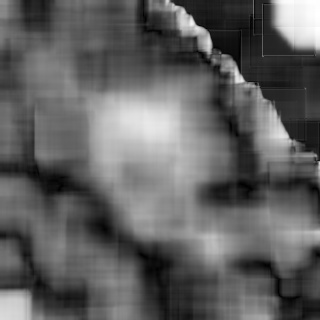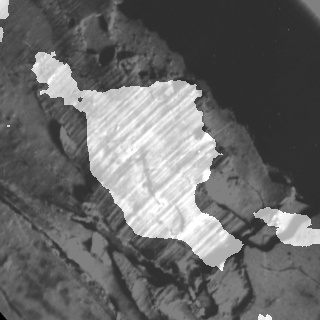OpenCV  4.5.5 Open Source Computer Vision
Anisotropic image segmentation by a gradient structure tensor

Prev Tutorial: Motion Deblur Filter

Next Tutorial: Periodic Noise Removing Filter

Compatibility OpenCV >= 3.0

## Goal

In this tutorial you will learn:

• what the gradient structure tensor is
• how to estimate orientation and coherency of an anisotropic image by a gradient structure tensor
• how to segment an anisotropic image with a single local orientation by a gradient structure tensor

## Theory

Note
The explanation is based on the books ,  and . Good physical explanation of a gradient structure tensor is given in . Also, you can refer to a wikipedia page Structure tensor.

### What is the gradient structure tensor?

In mathematics, the gradient structure tensor (also referred to as the second-moment matrix, the second order moment tensor, the inertia tensor, etc.) is a matrix derived from the gradient of a function. It summarizes the predominant directions of the gradient in a specified neighborhood of a point, and the degree to which those directions are coherent (coherency). The gradient structure tensor is widely used in image processing and computer vision for 2D/3D image segmentation, motion detection, adaptive filtration, local image features detection, etc.

Important features of anisotropic images include orientation and coherency of a local anisotropy. In this paper we will show how to estimate orientation and coherency, and how to segment an anisotropic image with a single local orientation by a gradient structure tensor.

The gradient structure tensor of an image is a 2x2 symmetric matrix. Eigenvectors of the gradient structure tensor indicate local orientation, whereas eigenvalues give coherency (a measure of anisotropism).

The gradient structure tensor $$J$$ of an image $$Z$$ can be written as:

$J = \begin{bmatrix} J_{11} & J_{12} \\ J_{12} & J_{22} \end{bmatrix}$

where $$J_{11} = M[Z_{x}^{2}]$$, $$J_{22} = M[Z_{y}^{2}]$$, $$J_{12} = M[Z_{x}Z_{y}]$$ - components of the tensor, $$M[]$$ is a symbol of mathematical expectation (we can consider this operation as averaging in a window w), $$Z_{x}$$ and $$Z_{y}$$ are partial derivatives of an image $$Z$$ with respect to $$x$$ and $$y$$.

The eigenvalues of the tensor can be found in the below formula:

$\lambda_{1,2} = \frac{1}{2} \left [ J_{11} + J_{22} \pm \sqrt{(J_{11} - J_{22})^{2} + 4J_{12}^{2}} \right ]$

where $$\lambda_1$$ - largest eigenvalue, $$\lambda_2$$ - smallest eigenvalue.

### How to estimate orientation and coherency of an anisotropic image by gradient structure tensor?

The orientation of an anisotropic image:

$\alpha = 0.5arctg\frac{2J_{12}}{J_{22} - J_{11}}$

Coherency:

$C = \frac{\lambda_1 - \lambda_2}{\lambda_1 + \lambda_2}$

The coherency ranges from 0 to 1. For ideal local orientation ( $$\lambda_2$$ = 0, $$\lambda_1$$ > 0) it is one, for an isotropic gray value structure ( $$\lambda_1$$ = $$\lambda_2$$ > 0) it is zero.

## Source code

You can find source code in the samples/cpp/tutorial_code/ImgProc/anisotropic_image_segmentation/anisotropic_image_segmentation.cpp of the OpenCV source code library.

## Explanation

An anisotropic image segmentation algorithm consists of a gradient structure tensor calculation, an orientation calculation, a coherency calculation and an orientation and coherency thresholding:

A function calcGST() calculates orientation and coherency by using a gradient structure tensor. An input parameter w defines a window size:

The below code applies a thresholds LowThr and HighThr to image orientation and a threshold C_Thr to image coherency calculated by the previous function. LowThr and HighThr define orientation range:

And finally we combine thresholding results:

## Result

Below you can see the real anisotropic image with single direction:Anisotropic image with the single direction

Below you can see the orientation and coherency of the anisotropic image:OrientationCoherency

Below you can see the segmentation result:Segmentation result

The result has been computed with w = 52, C_Thr = 0.43, LowThr = 35, HighThr = 57. We can see that the algorithm selected only the areas with one single direction.